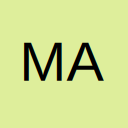# Ignore zero values in range for user defined function

• Hello,

I imported in my workbook a spline function that someone else has made, MySpline. MySpline takes two column ranges of equal lengths, one as

x-values you could say andone as y values, and one new x-value to be interpolated.

Now I am trying to make a template, so the input ranges will be fixed, fx A3:A13 and B3:B13. Users will insert their values in these two columns.

However, the inputs may vary. Fx let's say that one user only has data that fill A3:A9 and B3:B9. In that case, the remaining cells will be

empty and MySpline cannot handle that.

So I've tried different things. I've tried changing the VBA code of MySpline with something like

Code
``NewRange = InputRange(InputRange <> 0)``

I've tried the same following the method poste dby Kenneth here: VBA code to select cells with value greater than zero from a range.

I've tried

Code
``MySpline(\$A\$3:INDEX(A:A,COUNT(A:A)),\$B\$3:INDEX(B:B,COUNT(B:B)),C3)``

However, there are some headers at A1 and A2, so

Code
``INDEX(A:A,COUNT(A:A)) ``

doesn't give the correct last value in the column. It would give the value in A11.

I've tried

Code
``MySpline(\$A\$3:LOOKUP(2,1/(\$A\$3:\$A\$13<>""),\$A\$3:\$A\$13)),\$B\$3:LOOKUP(2,1/(\$B\$3:\$B\$13<>""),\$B\$3:\$B\$13)),B3``

But I figured out that INDEX and LOOKUP don't work together.

I've tried making the cells be empty (see Return empty cells and here - the part with the "suicide cells") for some reason the "suicide cell function" doesn't work for me.

Now I've run out of ideas...Is there anyone who could help? Either with a better idea or improving my previous ideas.

I can do this with a button and VBA, however I would prefer a solution without no user interaction other than the input, such that the user inputs some data, MySpline does the math.

NB: I am not exactly sure if this is the right place to post this and if I'm using the "code" function correctly as I am new here...

• Hi, I can unfortunately not do thatI tried to write a detailed description hoping it would describe enough

• You could use:

\$A\$3:INDEX(\$A\$3:\$A\$13,COUNT(\$A\$3:\$A\$13))

Rory
Theory is when you know something, but it doesn’t work. Practice is when something works, but you don’t know why. Programmers combine theory and practice: nothing works and they don’t know why

## Participate now!

Don’t have an account yet? Register yourself now and be a part of our community!